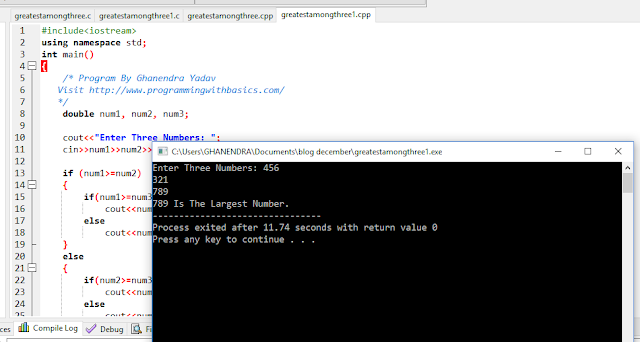# C++ Program To Find Greatest Among Three Numbers

Problem :- Write A C++ Program To Find Greatest Among Three Numbers .Take three numbers from user and print the greatest number among them ex :- three numbers are 10 ,20 ,30 then 30 is greatest show 30 in output screen .

Logic :- For this problem there is a simple logic that take all three numbers and choose one number and compare that number to rest of the two number is number is greatest then print else choose second number and repeat the previous process if greatest then print second number is greatest else go to third number and repeat the same process .We are taking a Example and check all the process Step by Step

Example :- We are taking a Three number 55,65,75 as Input

Step 1 :- Select first number 55 and compare to 65 and 75 as we know 55 is not greater so control transfer to second condition .

Step 2 :- Now choose Second number 65 and compare to 55 and 75 as we know 65 is greater that 55 but not greater than 75 so control transfer to Third condition .

Step 3 :- Now choose Third number 75 and compare to 65 and 55 as we know 75 is greater compare both number 55 and 65 .Now we have a greatest number then print the number

Step 4 :- This is a Special Step if all condition didn't Match then All Number are equal .Example :- 50 ,50 ,50  then control goes to last condition and program  Output is "All Are Equal "

Solution :-

Method 1:-

#include<iostream>
using namespace std;
int main()
{
Visit http://www.programmingwithbasics.com/
*/
double num1, num2, num3;

cout<<"Enter Three Numbers: \n";
cin>>num1>>num2>>num3;

if (num1 > num2 && num1 > num3)
cout<<"\nGreatest Number Is :"<<num1;

else if (num2 > num3 && num2 > num1)
cout<<"\nGreatest Number Is :"<<num2;

else if (num1 > num1 && num3 > num2)
cout<<"\nGreatest Number Is :"<<num1;

else if ((num1==num2) && (num2==num3) && (num3==num1))
cout<<"\nAll Are Equal";

}

Method 2:-

#include<iostream>
using namespace std;
int main()
{
Visit http://www.programmingwithbasics.com/
*/
double num1, num2, num3;

cout<<"Enter Three Numbers: ";
cin>>num1>>num2>>num3;

if (num1>=num2)
{
if(num1>=num3)
cout<<num1<<" Is The Largest Number. ";
else
cout<<num3<<" Is The Largest Number. ";
}
else
{
if(num2>=num3)
cout<<num2<<" Is The Largest Number. ";
else
cout<<num3<<" is the largest number.";
}

return 0;

}

Output:-Previous Post
Next Post

#### post written by: Ghanendra Yadav

Hi, I’m Ghanendra Yadav, SEO Expert, Professional Blogger, Programmer, and UI Developer. Get a Solution of More Than 500+ Programming Problems, and Practice All Programs in C, C++, and Java Languages. Get a Competitive Website Solution also Ie. Hackerrank Solutions and Geeksforgeeks Solutions. If You Are Interested to Learn a C Programming Language and You Don't Have Experience in Any Programming, You Should Start with a C Programming Language, Read: List of Format Specifiers in C.